# Dielectric function tools¶

New in version 0.7.

## Number of effective electrons¶

New in version 0.7.

The Bethe f-sum rule gives rise to two definitions of the effective number (see [Egerton2011]):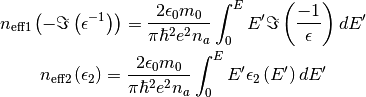where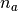is the number of atoms (or molecules) per unit volume of the sample,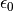is the vacuum permittivity,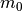is the elecron mass and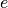is the electron charge.

The get_number_of_effective_electrons() method computes both.

## Compute the electron energy-loss signal¶

New in version 0.7.

The get_electron_energy_loss_spectrum() “naively” computes the single-scattering electron-energy loss spectrum from the dielectric function given the zero-loss peak (or its integral) and the sample thickness using: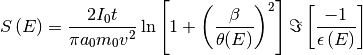where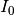is the zero-loss peak integral,the sample thickness,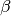the collection semi-angle and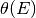the characteristic scattering angle.